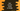# How to convert string to integer in dart## Convert string to integer in Dart:

In this post, we will learn how to convert one string to integer in Dart. Also, we will check different examples with different integers.

### Convert string to int in dart:

For converting string to int in dart, we can use either parse or tryParse method. Let’s try them one by one:

### String to int using parse:

parse method is defined in integer class in Dart. This is a static method and it is defined as below:

``int parse(String strValue, {int? radix})``

Here, strValue is the string value of the integer variable and radix is an optional value.

By default, radix is 10 i.e. for decimal conversions. But we can give it a value in range 2..36.

The strValue is the string value that we need to convert to integer. It must not be null. For invalid inputs, it throws FormatException.

### Example of parse:

Below are a couple of different use cases of parse:

``````void main() {
int firstInt = int.parse('1');
int secondInt = int.parse('10000');
int thirdInt = int.parse('-100');
int fourthInt = int.parse('+100');

print("firstInt : \$firstInt");
print("secondInt : \$secondInt");
print("thirdInt : \$thirdInt");
print("fourthInt : \$fourthInt");
}``````

It will print:

``````firstInt : 1
secondInt : 10000
thirdInt : -100
fourthInt : 100``````

So, as you can see here, we can pasrse strings that defines an integer and even if it has a positive or negative sign at front.

### Exceptions in parse:

parse will throw exception for invalid inputs. For example:

``````void main() {
try {
int firstInt = int.parse('99999999999999999999');
} on FormatException {
print("FormatException for firstInt");
}
try {
int secondInt = int.parse('2.5');
} on FormatException {
print("FormatException for secondInt");
}
try {
int thirdInt = int.parse('2,3');
} on FormatException {
print("FormatException for thirdInt");
}
try {
int fourthInt = int.parse('ten');
} on FormatException {
print("FormatException for fourthInt");
}
try {
int fifthInt = int.parse(null);
} on ArgumentError {
print("Invalid argument exception for fifthInt");
}
}``````

All of these cases will throw exception.

• For firstInt, we are trying to parse a value more than 2^63 - 1. It should be in the range of -2^63 to 2^63 - 1
• For secondInt, parsing doesn’t support any string that contains any separator character.
• thirdInt is same as secondInt.
• For fourthInt, we are trying to parse a string.
• For fifthInt, we are trying to parse null. Note that it throws ArgumentError, not an exception. It throws an error like below:

If you run the above problem, it will print the below output:

``````FormatException for firstInt
FormatException for secondInt
FormatException for thirdInt
FormatException for fourthInt
Invalid argument exception for fifthInt``````

### Using tryParse:

tryParse is better than parse. It handles the exceptions. For example,

``````void main() {
print(int.tryParse('99999999999999999999'));

print(int.tryParse('2.5'));

print(int.tryParse('2,3'));

print(int.tryParse('ten'));

print(int.tryParse(null));
}``````

It will print:

``````null
null
null
null
Unhandled exception:
Invalid argument(s): The source must not be null``````

It returns null for all except the last one where we are passing null. So, tryParse doesn’t work for null.